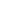| lesson material | material summary | questions and answers | definitions | types and examples | other information | materi pelajaran | ringkasan materi | pertanyaan dan jawaban | definisi | jenis-jenis dan contoh-contoh | informasi lainnya |

# Learning Javascript: Know 5 Types of Repeat Forms in JavascriptWhat will you do when you are told to print a sentence over and over?
For example:
"Please show the sentence `"Tutorial Javascript!"`on my website 10 times"
Maybe you can write it with functions `document.write()`10 times like this:
``````document.write("<p>Tutorial Javascript!</p>");
document.write("<p>Tutorial Javascript!</p>");
document.write("<p>Tutorial Javascript!</p>");
document.write("<p>Tutorial Javascript!</p>");
document.write("<p>Tutorial Javascript!</p>");
document.write("<p>Tutorial Javascript!</p>");
document.write("<p>Tutorial Javascript!</p>");
document.write("<p>Tutorial Javascript!</p>");
document.write("<p>Tutorial Javascript!</p>");
document.write("<p>Tutorial Javascript!</p>");
``````
The result: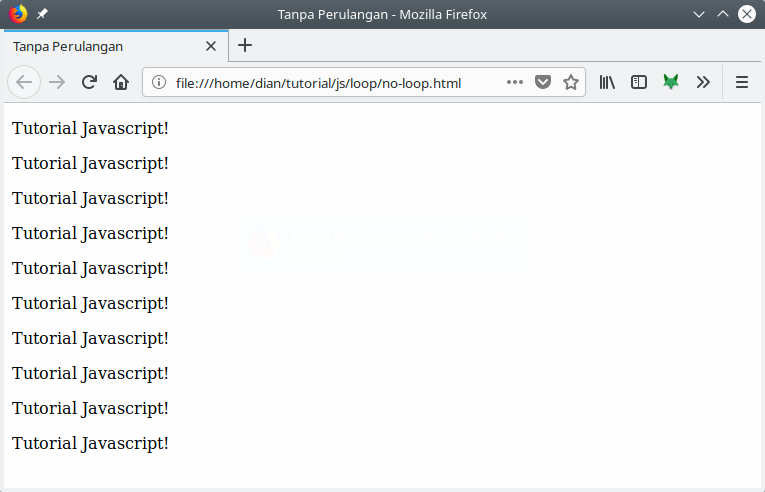Can it be like this?
Yes, it's okay.
But…
What if later he wants to display as many `1000`times.
Must be tired don't type it.
Therefore, we must use repetition .

Repetition will help us execute repeated code, no matter how much we want.
There are five types of looping in Javascript. In general, this loop is divided in two.
Namely: counted loop and uncounted loop .
Difference:
• Counted Loop is a clear loop and certainly a lot of repetitions.
• Whereas Uncounted Loop , is a loop that is unclear how many times he has to reply .
Repetition included in Counted Loop :
1. Repeat For
2. Foreach loop
3. Repeat Repeat
Repetition included in Uncounted Loop :
1. While repetition
2. Do / While repetition
Let's discuss them one by one…

## 1. Repeat For Javascript

Repetition `for`is a loop that is included in the couted loop , because it's clear how many times it will repeat.
The code is like this:
``````for(let i = 0; i < 10; i++){
document.write("<p>Perulangan ke-" + i + "</p>")
}
``````
What needs to be considered is the condition in parentheses after the word `for`.
This condition will determine:
• The count will start from `0``i = 0`);
• How many counts? Arrived `i < 10`;
• Then in each loop `i`will increase `+1``i++`).
The variable `i`in repetition `for`functions to store the count value.
So each loop is done, the value `i`will always increase by one. Because we determine it in part `i++`.
This is the output: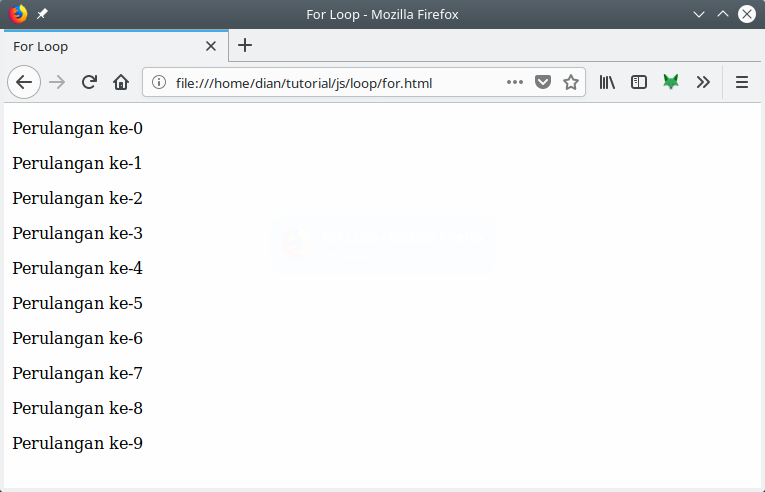Does the variable name always have to be `i`?
Not.
We can also use other names.
For example:
``````for(counter = 0; counter < 50; counter+=2){
document.write("<p>Perulangan ke-"+counter+"</p>");
}
``````
In this example, we do a loop starting from zero `0`Then in each loop the value of the variable `couter`will be added to 2 ( `counter+=2`).
The result: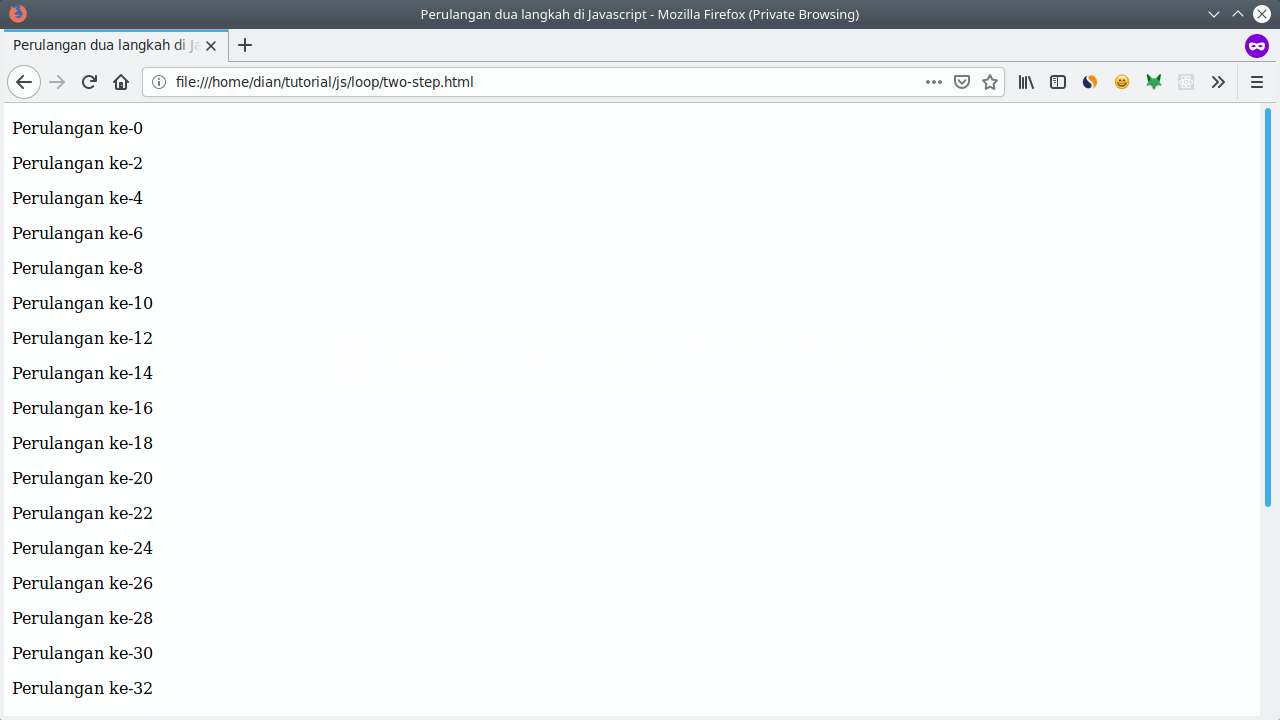What if the loop counter starts from the bigger akanga to the smallest one?
We usually make this when we want to count down ...
The trick is easy.
We just fill in the counter value with the greatest value.
For example we will start counting from `10`to `0`.
Then the value `counter`, we fill it initially with `10`.
Then in the comparison conditions, we give `counter > 0`This means that the loop will be carried out as long as the counter value is greater than `0`.
Then we subtract ( `-1`) the counter value in each loop ( `counter--`).
``````for(counter = 10; counter > 0; counter--){
document.write("<p>Perulangan ke "+counter+"</p>");
}
``````
The result: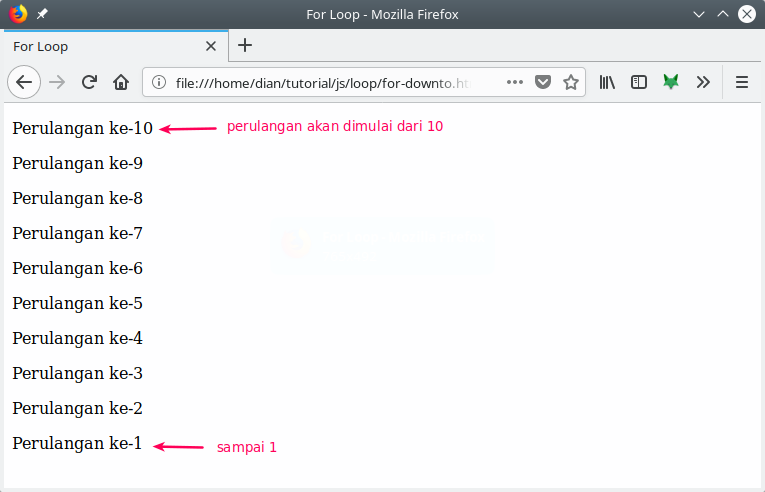Why not to zero ( `0`)?
Because of the conditions we give `counter > 0`If it is `counter`worth `0`, then this condition will become `false`.
Unless we use a larger operator equal to ( `>=`), then if it is `counter`worth `0`, the condition will be `true`.

## 2. While repetition is in Javascript

Repetition `while`is a loop that is included in the uncounted loop loop .
Repetition `while`can also be a counted loop loop by providing a counter in it.
To understand this loop ...
... let's see an example:
``````var ulangi = confirm("Apakah anda mau mengulang?");
var counter = 0;

while(ulangi){
var jawab = confirm("Apakah anda mau mengulang?")
counter++;
if(jawab == false){
ulangi = false;
}
}

document.write("Perulangan sudah dilakuakn sebanyak "+ counter +" kali");
``````
Can be simplified to:
``````var ulangi = confirm("Apakah anda mau mengulang?");
var counter = 0;

while(ulangi){
counter++;
ulangi = confirm("Apakah anda mau mengulang?");
}

document.write("Perulangan sudah dilakuakn sebanyak "+ counter +" kali");
``````
The result: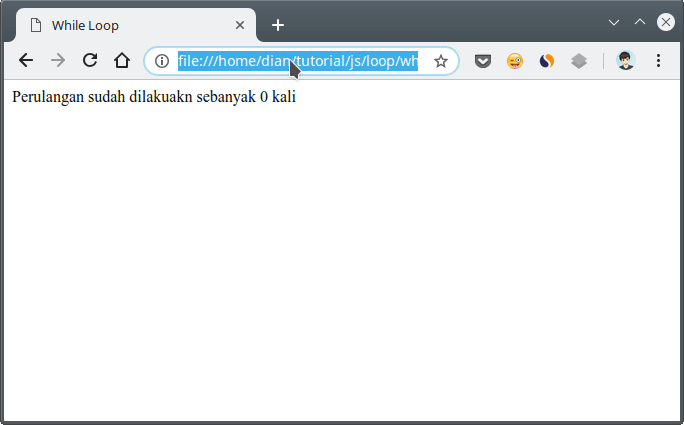Consider the code block `while`:
``````while(ulangi){
counter++;
ulangi = confirm("Apakah anda mau mengulang?");
}
``````
There ... Repetition will occur during the `ulangi` value variable `true`.
Then we use the function `confirm()`to display the confirmation dialog.
As long as we choose Ok on the confirmation dialog, the variable `ulangi`will continue to be of value `true`.
But if we select Cancel , the variable `ulangi` will be worth `false`.
When a variable is `ulangi`valued `false`, the loop will be stopped.

## 3. Repeat Do / While in Javascript

Repetition is the `do/while`same as repetition `while`.
Difference:
Repetition `do/while`will repeat as many `1`times first, then check the conditions in parentheses `while`.
Form it like this:
``````do {
// blok kode yang akan diulang
} while (<kondisi>);
``````
So the difference is:
Repetition `do/while`will check the condition behind (after repeating), while it `while`will check the condition in front or the beginning (before repeating).
Let's look at an example:
``````var ulangi = confirm("Apakah anda mau mengulang?");;
var counter = 0;

do {
counter++;
ulangi = confirm("Apakah anda mau mengulang?");
} while(ulangi)

document.write("Perulangan sudah dilakuakn sebanyak "+ counter +" kali");
``````
The example is the same as the example of repetition `while`.
During the first iteration, try canceling the loop by selecting Cancel .
Then the result: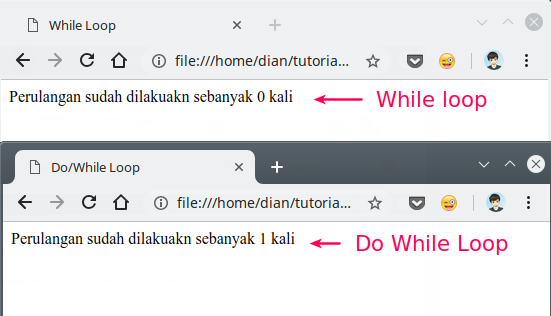## 4. Foreach recurrence in Javascript

Loops are `foreach`usually used to print items in an array.
This loop is included in the counted loop loop , because the number of repetitions will be determined by the length of the array.
There are two ways to use looping `foreach` in JavaScript:
1. Using `for`with operators `in`;
2. Use method `forEach()`.
Example:
Here is the "foreach" loop without using the operator `in`:
``````var languages = ["Javascript", "HTML", "CSS", "Typescript"];

for(i = 0; i < languages.length; i++){
document.write(i+". "+ languages[i] + "<br/>");
}
``````
This looping can be made even simpler by using an operator `in`like this:
``````var languages = ["Javascript", "HTML", "CSS", "Typescript"];

for(i in languages){
document.write(i+". "+ languages[i] + "<br/>");
}
``````
The result: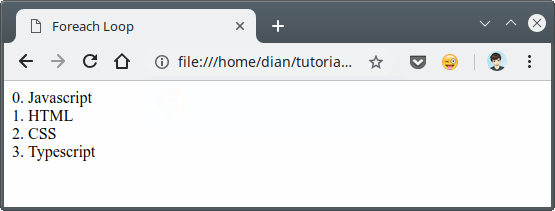The second way to make looping `foreach`is to use `forEach()`an array method .
Example:
``````// kita punya array seperti berikut
var days = ["Senin", "Selasa", "Rabu", "Kamis", "Jum'at", "Sabtu", "Minggu"];

// Kemudian kita tampilkan semua hari
// dengan menggunakan method foreach
days.forEach(function(day){
document.write("<p>" + day + "</p>");
});
``````
The method `forEach()`has a parameter in the form of a callback function Actually we can also use arrow functions like this:
``````// kita punya array seperti berikut
var days = ["Senin", "Selasa", "Rabu", "Kamis", "Jum'at", "Sabtu", "Minggu"];

// Kemudian kita tampilkan semua hari
// dengan menggunakan method foreach
days.forEach((day) => {
document.write("<p>" + day + "</p>");
});
``````
The result: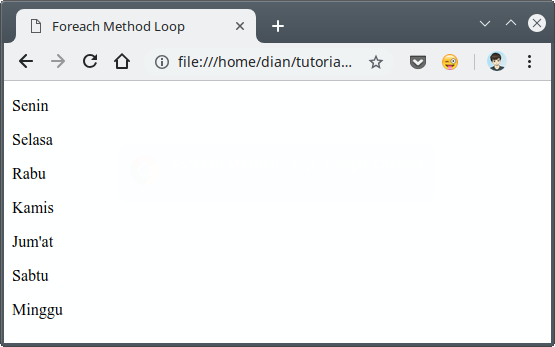An explanation of the arrow function, will be discussed in the "Javascript Tutorial: Understanding Functions in JavaScript" .

## 5. Repeat with repeat () methods

Repeat with methods or functions `repeat()` included in counted loop loops .
This function is specifically used to repeat a text (string).
It can be said that:
This is short of repetition `for`.
Example:
If we use looping `for`:
``````for( let i = 0; i < 100; i++){
document.write("Ulangi kalimat ini!");
}
``````
If we use the function `repeat()`:
``````document.write("Ulangi kalimat ini! ".repeat(100));
``````
The result: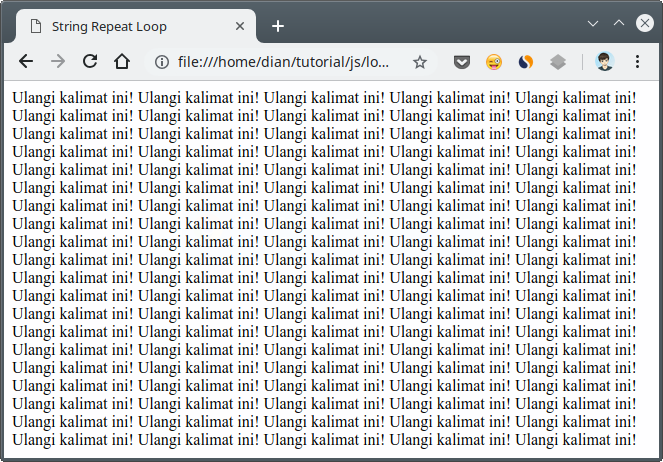## 6. Bonus: Nested Repetition

In looping blocks, we can also make repetitions.
This is called nested loop or repetition in adventure.
Let's look at an example:
``````for(let i = 0; i < 10; i++){
for(let j = 0; j < 10; j++){
document.write("<p>Perulangan ke " + i + "," + j + "</p>");
}
}
``````
The result: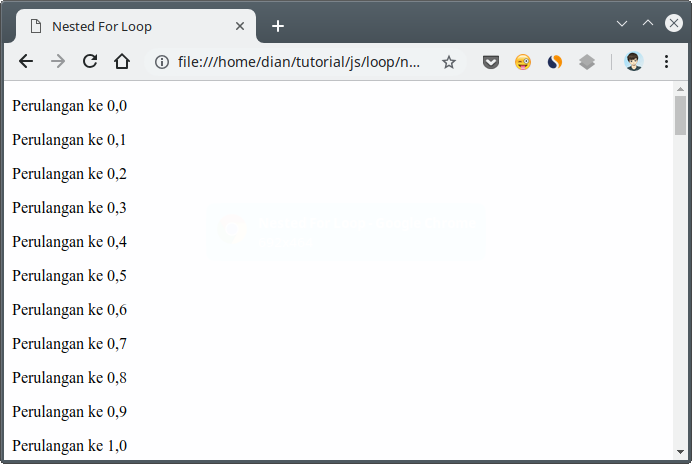In that loop, we use two repetitions `for`.
The first loop uses variables `i`as counters , while the second emperor uses variables `j`as counters .
Another example:
``````var ulangi = confirm("apakah anda ingin mengulang?");
var counter = 0;

while (ulangi) {
counter++;
var bintang = "*".repeat(counter) + "<br>";
document.write(counter + ": " + bintang);
ulangi = confirm("apakah anda ingin mengulang?");
}
``````
The result: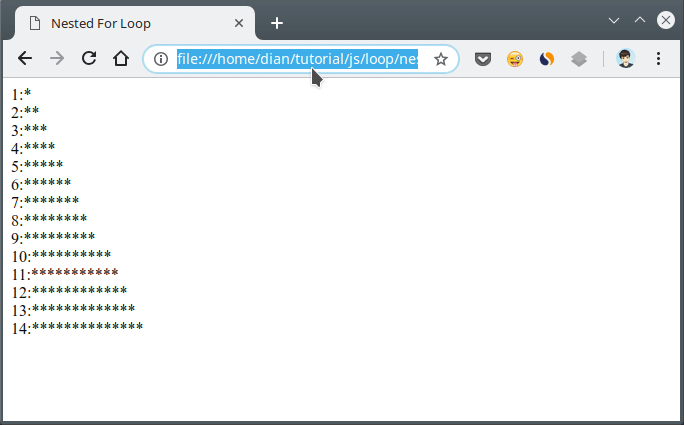Later, we will use many nesting loops when working with two-dimensional arrays.
We can make as many extensions as possible in the other loop.
However, this needs to be considered:
The more repetitions, the computer will process it the longer.
Of course this will make your website or application run slowly.
Therefore, use looping wisely##### 0 Komentar untuk "Learning Javascript: Know 5 Types of Repeat Forms in Javascript"

Silahkan berkomentar sesuai artikel

Template By Kunci Dunia